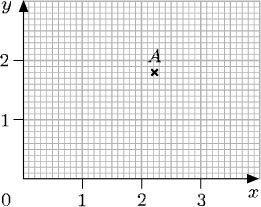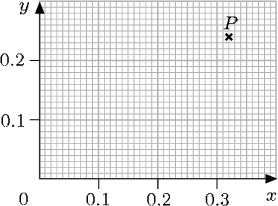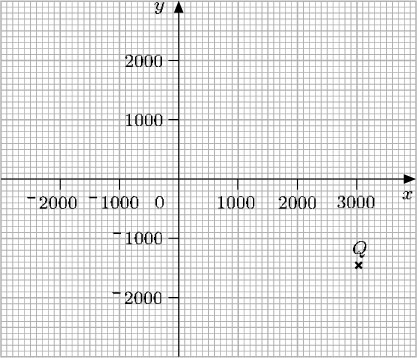Science, Maths & Technology

### Become an OU studentDiagrams, charts and graphs

Start this free course now. Just create an account and sign in. Enrol and complete the course for a free statement of participation or digital badge if available.

# 3.3 Decimal and fraction coordinates

Where necessary, the coordinates of a point can be specified by using decimals or fractions. However, locating points whose coordinates are not whole numbers requires more precision when reading along the axes. For example, here the coordinates of the point A are (2.2, 1.8).A point P with coordinates (0.32, 0.24) would be particularly difficult to plot accurately on the axes above because of the scales used. You can only plot the point approximately. But, if a larger scale were used for the axes, it would be quite easy to plot P with reasonable accuracy, as shown below.Similarly, to plot points with very large coordinates, such as a point Q with coordinates (3020, −1450), a very different scale would be needed.Notice that, even using scales like these, it is impossible to plot points such as Q exactly.

Mathematical coordinate grids are used for maps, plans, geometric diagrams and also for graphs. For the first three of these, the scales on the axes of the grid must be the same (otherwise shapes would be distorted and diagonal distances would be incorrect). For graphs, the scales on the axes need not be the same – in fact, often the two scales represent very different things.